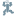# Kakuro Light

• Currently 4.3/5
• 1
• 2
• 3
• 4
• 5

What do you get when you mix the logic of sudoku with the clue-solving challenge of a crossword? Kakuro Light is Conceptis Puzzles' latest entry in their miniature puzzle series, but don't let the tiny package fool you. These puzzles really add up to a tricky challenge!

### Walkthrough Guide

(Please allow page to fully load for spoiler tags to be functional.)

Kakuro Tips:

• One important tip for quickly solving Kakuro puzzles is to remember minimum and maximum values for strings of numbers of a certain length. For example, if a string is two digits long, and has a target sum of 3, you know the numbers in that string must be 1 and 2 in some order. Similarly, if the target sum is 4, the numbers must be 1 and 3 (2 and 2 would be illegal). On the other end of the spectrum, if a string of two numbers has a target sum of 17, you know it must contain a 9 and 8, while a sum of 16 must have 9 and 7. For a full list of sums you can automatically assume, click this spoiler tag:

For a two-box string:

• 3 = 1+2

• 4 = 1+3

• 17 = 9+8

• 16 = 9+7

For a three-box string:

• 6 = 1+2+3

• 7 = 1+2+4

• 24 = 7+8+9

• 23 = 6+8+9

For a four-box string:

• 10 = 1+2+3+4

• 11 = 1+2+3+5

• 30 = 6+7+8+9

• 29 = 5+7+8+9

For a five-box string (getting the hang of this yet?):

• 15 = 1+2+3+4+5

• 16 = 1+2+3+4+6

• 35 = 5+6+7+8+9

• 34 = 4+6+7+8+9

For a six-box string:

• 21 = 1+2+3+4+5+6

• 22 = 1+2+3+4+5+7

• 39 = 4+5+6+7+8+9

• 38 = 3+5+6+7+8+9

For a seven-box string:

• 28 = 1+2+3+4+5+6+7

• 29 = 1+2+3+4+5+6+8

• 42 = 3+4+5+6+7+8+9

• 41 = 2+4+5+6+7+8+9

For an eight-box string: Rather than typing out every combination, here's the trick to use here: Take a look at the target sum, and subtract it from 45 (which is the sum of all of the digits from 1 to 9). Whatever the difference is is the number to omit from your string. For example, if the sum is 43, your string is 1+3+4+5+6+7+8+9, omitting the 2.
For a nine-box string: USE ALL THE NUMBARS! (It's best if you imagine a stick-figure version of me waving a 9 in the air as I shout that.)

• Look for spots where any of the given patterns cross as starting points. For example, if you see two strings of two boxes with the sums of 16 and 17 intersect, you know the shared box has a 9.

• Even without using the above list of givens, you can also make good progress by looking for the intersections of high and low sums. For example, if you see two strings of two boxes with the sums 14 and 6 intersect, you know the shared box has a 5, because it's the highest possible digit for the sum of 6, and also the lowest possible digit for the sum of 14.

• Don't forget to eliminate possibilities! If you click the smaller shaded box in each square, you can write a small number in the box to remind you of what's possible in that square or not.

• Don't be afraid of good-old-fashioned trial and error! Again, use the tiny numbers to assist you on guesswork.

Kakuro Light Solutions

Levels 1 (8x8)

Puzzle 1:

Puzzle 2:

Puzzle 3:

Puzzle 4:

Puzzle 5:

Puzzle 6:

Puzzle 7:

Puzzle 8:

Puzzle 9:

Puzzle 10:

Level 2 (9x9)

Puzzle 1:

Puzzle 2:

Puzzle 3:

Puzzle 4:

Puzzle 5:

Puzzle 6:

Puzzle 7:

Puzzle 8:

Puzzle 9:

Puzzle 10:

Level 3 (10x10)

Puzzle 1:

Puzzle 2:

Puzzle 3:

Puzzle 4:

Puzzle 5:

Puzzle 6:

Puzzle 7:

Puzzle 8:

Puzzle 9:

Puzzle 10:

At last!

I've been waiting forever for conceptis to release a Kakuro edition of the conceptis lite seriesSeptember 15, 2011 10:03 AM

YESSSS

YESSSSSS

Finally a way to play Kakuro on my computer, without the aid of the Guardian's puzzle section and Paint !

There is only a limited amount, though. >:

Please consider creating a Casual Gameplay account if you're a regular visitor here, as it will allow us to create an even better experience for you. Sign-up here!
• You may use limited HTML tags for style:
(a href, b, br/, strong, em, ul, ol, li, code, spoiler)
HTML tags begin with a less-than sign: < and end with a greater-than sign: >. Always. No exceptions.
• To post spoilers, please use spoiler tags: <spoiler> example </spoiler>
If you need help understanding spoiler tags, read the spoiler help.
• No link dropping, no domains as names; do not spam, and do not advertise! (rel="nofollow" in use)

### HELP Jayisgames.com